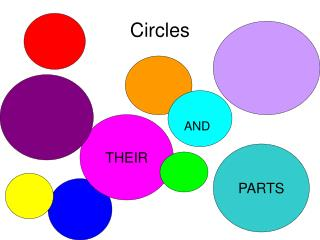# Circles - PowerPoint PPT PresentationDownload PresentationCircles

CirclesDownload Presentation## Circles

- - - - - - - - - - - - - - - - - - - - - - - - - - - E N D - - - - - - - - - - - - - - - - - - - - - - - - - - -
##### Presentation Transcript

1. Circles AND THEIR PARTS

2. D I A M E T E R CHORD Radius A line outside the circle and touching the circle at one point. TANGENT Tangent lines intersecting a diameter or radii at the point of tangency are perpendicular

3. CENTRAL ANGLES A Central Angles- angles whose vertex is at the center of the circle 1200 The intercepted arc of a central angle is equal to the measure of the central angle B ARC AB = 1200 C

4. Inscribed Angle 100o A An inscribed angle is an angle with its vertex ”on” the circle, formed by two intersecting chords Inscribed angle = ½ intercepted Arc C O <ABC is an inscribed angle. Its intercepted arc is minor arc from A to C, therefore m<ABC = 500 B

5. Inscribed Quadrilateral • A quadrilateral can be inscribed in a circle if and only if its opposite angles are supplementary 140˚ 70˚ 120˚ 40˚

6. A Triangle inscribed in a circle • A triangle inscribed in a semi-circle , having a hypotenuse as a diameter is a right triangle. 60˚ 30˚ P

7. Tangent Chord Angle C B An angle formed by an intersecting tangent and chord with the vertex “on “ the circle. 120o Tangent Chord Angle = ½ intercepted arc <ABC is an angle formed by a tangent and chord. Its intercepted arc is the minor arc from A to B, therefore m<ABC = 60o A

8. Angles formed by intersecting chords (not diameters) 80 When two chords intersect “inside” a circle, four angles are formed. At the point of intersection, two sets of vertical angles can be seen in the corners of the X that is formed on the picture. Remember vertical angles are equal. A B E 60 50 D C Angle formed inside by two chords= ½ sum of the intercepted arcs m< AEB = ½(80 +170) = 1/(250) = 125 170

9. Angle Formed Outside of a Circle by the Intersection of: • “Two Tangents” A B 150o The angle is ½ of the difference of the two arcs formed by the tangents. >ABC = ½(210 – 150) = 30˚ D C 2100

10. “Two Secants” C A 20o An angle formed by two secant on the outside of the circle . The measure of the angle is ½ the difference of the intersected arcs. <ACB = ½(95 – 20) = 37.5˚ B D 95o E